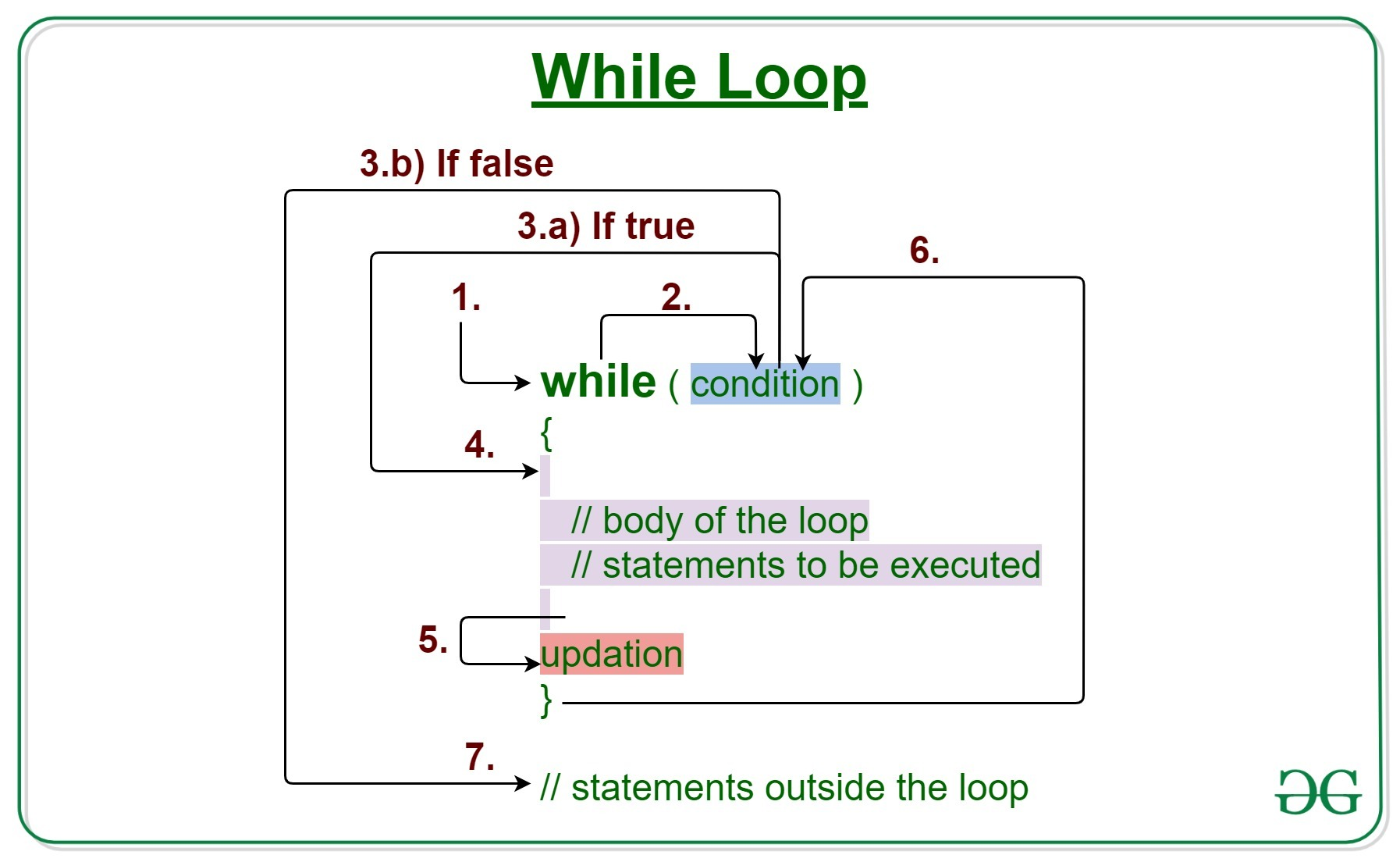# Java Program to Find Sum of Natural Numbers Using While Loop

• Difficulty Level : Basic
• Last Updated : 14 Jun, 2022

While loop arises into play where beforehand there is no conclusive evidence that how many times a loop is to be executed. This is the primary reason as there is no strict tight bound over how many numbers the sum is to be evaluated. Situations in which the output can be displayed using test condition abiding hardcoded outputs by simply running the programs, while the loop is taken for consideration.Syntax:

```while (test_expression)
{
// statements

update_expression;
}```

Sum: The sum of natural numbers from ‘1’ to ‘n’ can be mathematically written where n represents the number of numbers entered by the user or to be evaluated. Using the principle of mathematical induction above formula equals:

`1 + 2 + 3 + 4 + 5 + ...+ (n-2) + (n-1) + n = [n(n+1)]/2`

Illustration: Suppose the sum of 10 natural numbers is to be calculated then by above formula 55 should be the output.

```Input      : 5
Processing :  1 + 2 + 3+ 4 + 5
Output     : 15```

Approach: Using a While loop where a condition is passed as a parameter in a while statement which is referred to as a ‘test condition’.

1. Test Expression: In this expression, we have to test the condition. If the condition evaluates to true then we will execute the body of the loop and go to update expression. Otherwise, we will exit from the while loop.
Example: i ≤ 10
2. Update Expression: After executing the loop body, this expression increments/decrements the loop variable by some value.
Example: i++;

Algorithm: for the sum of natural numbers using while loop is as follows

• Initializing n=10,sum=0,i=1; //where n is the number till the user want sum
• If the natural number to be processed holds the test condition, compute the below steps, and if fails display the current sum as the final sum.
• The current sum is updated as the test condition holds true to the final sum.
• Increment the variable to move to the next natural number and if the test condition holds true, update the existing sum.
• Display the sum
• Terminate

Implementation:

## Java

 `// Java program to show sum of natural numbers``// using the while loop` `import` `java.util.*;` `class` `GFG {` `    ``public` `static` `void` `main(String[] args)``    ``{``        ``int` `n = ``10``, sum = ``0``, i = ``1``;` `        ``/* While loop*/` `        ``// Test condition``        ``while` `(i <= n) {` `            ``/* Statements to execute */` `            ``// Update the current sum till``            ``// test condition holds true``            ``sum = sum + i;` `            ``// Increment the variable counter``            ``// or jumping to next natural number``            ``i++;``        ``}` `        ``// Print the sum``        ``System.out.println(``            ``"Sum of natural numbers using while loop is:"``            ``+ ``" "` `+ sum);``    ``}``}`

Output

`Sum of natural numbers using while loop is: 55`

Time Complexity: O(n)

Auxiliary Space: O(1)

My Personal Notes arrow_drop_up